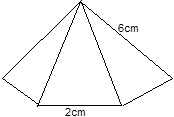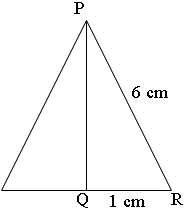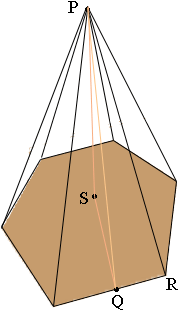SEARCH HOMEMath Central Quandaries & QueriesQuestion from carol, a student: i have been set a question that gives a regular hexagon and each of the sloping sides is 6cm from a corner of a base to the apex(point). it also has 2cm sides it looks sort of like this: /\6cm 2cm how do i find its height when only given those measurements?When we asked for clarification Carol sent this drawingHi Carol,

It looks like you have a pyramid constructed on a base which is a regular hexagon. Look at one of the triangles that form the sides.Q is the midpoint of one of the sides of the base so angle PQR is a right triangle. Hence you can use Pythagoras' theorem to find the length |PQ|.

Now look at the three dimensional pyramid again.S is at the centre of the hexagonal base so |PS| is the height of the pyramid. Angle PSQ is a right angle so again you can use Pythagoras' theorem to find |PS| if you can first find |SQ|.

|SQ| is half the width of the hexagon which you can find using our response to an earlier question.

PennyMath Central is supported by the University of Regina and The Pacific Institute for the Mathematical Sciences.